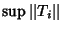## Uniform Boundedness Principle

A ``pointwise-bounded'' family of continuous linear Operators from a Banach Space to a Normed Space is ``uniformly bounded.'' Symbolically, ifis Finite for eachin the unit Ball, thenis Finite. The theorem is also called the Banach-Steinhaus Theorem.

References

Zeidler, E. Applied Functional Analysis: Applications to Mathematical Physics. New York: Springer-Verlag, 1995.